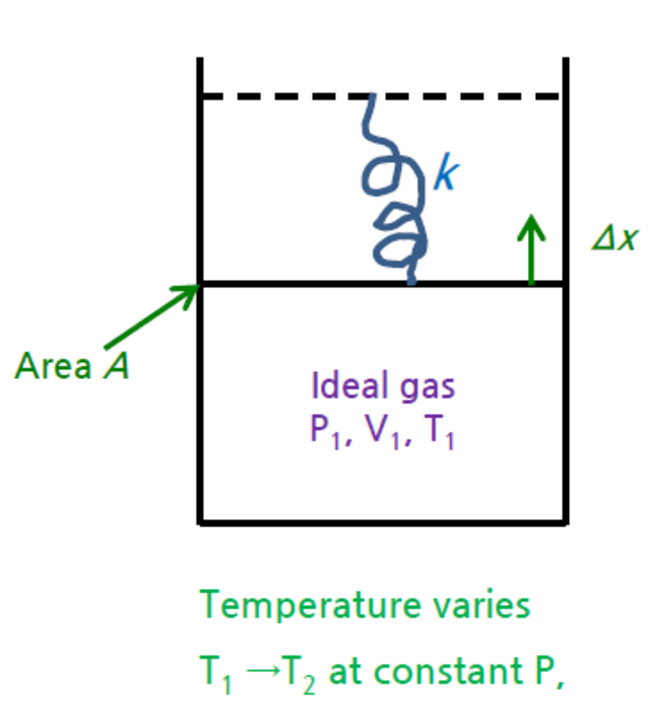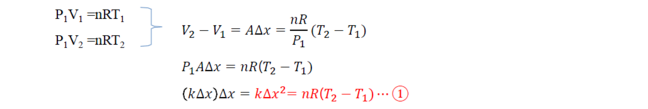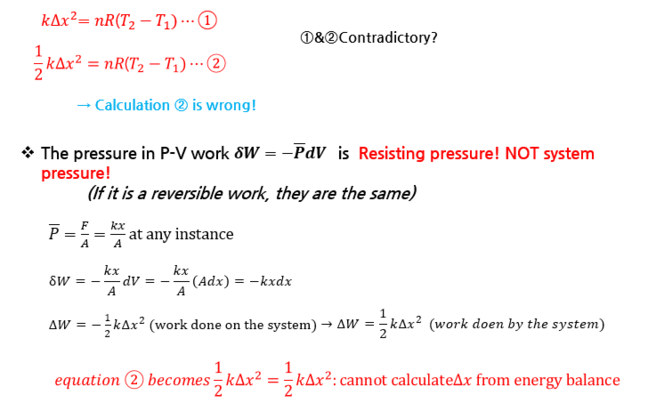## Want to keep learning?

This content is taken from the Hanyang University's online course, Thermodynamics in Energy Engineering. Join the course to learn more.
2.7

# Example 2: Equation of state for ideal gas

### Example : In a gas-spring systemAn ideal gas is contained in a cylinder. Initially the pressure, volume and temperature were P1, V1, and T1. A spring with a spring constant k attaches to the piston as the figure.The area of piston is A.The gas expands against the spring by increasing temperature to at constant pressure T2.What is the displacement? Let final displacement as Δx

#### We can address this problem by two approaches; the force balances or the energy balances.

① Force balance at the piston

The force exerted by the spring due to the displacement is balanced     with the gas pressure

$$F$$=$$kΔx$$=$$P$$gas$$A$$=$$P$$1$$A$$
Ideal gas law holds for all ideal gas② Energy balance
Work done by the gas = Energy stored in spring